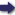(London :  Kegan Paul, Trench, Trübner & Co.,  1910.)

 Tools

## Search this bookPrev Page 32 Next``` 32 ALBERUNTS INDIA. i.e. 1,080,000, and get the product 26,423,470,080,000. These are the years which have elapsed before the present kcdpa. We further multiply the latter number by 12, so as to get months, viz. 317,081,640,960,000. We write down this number in two different places. In the one place, we multiply it by the number of the ad,himdsct months of a caturyuga, i.e. 1,593,336, or a corresponding number which has been mentioned in the preceding, and we divide the product by the num¬ ber of the solar months of a caturyuga, i.e. 51,840,000. The quotient is the number of adhimdsa months, viz. 9>745,709,75o,784. This number we add to the number written in the second place, and get the sum of 326,827,350,710,784. Multiplying this number by 30, we get the product 9,804,820,521,323,520, viz. lunar days. This number is again written down in two different places. In the one place we multiply it by the 'Anardtra of a caturyuga, i.e. the difference between civil and lunar days, and divide the product by the lunar days of a catur¬ yuga. Thus we get as quotient 153,416,869,240,320, i.e. unardtra days. We subtract this number from that one written in the second place, and we get as remainder 9,651,403,652,083,200, i.e. the days which have elapsed of the life of Brahman before the present kalpa, or the days of 6068 kcdpas, each kcdpa having 1,590,541,142,400 days. Dividing this sum of days by 7, we get no remainder. This period of time ends with a Saturday, and the present kcdpct commences with a Sunday. This shows that the beginning of the life of Brahman too was a Sunday. Of the current kcdpct there have elapsed six manvan¬ taras, each of 72 caturyugas, and each caturyuga of 4,320,000 years. Therefore six manvantaras have 1,866,240,000 years. This number we compute in the ```Prev Page 32 Next